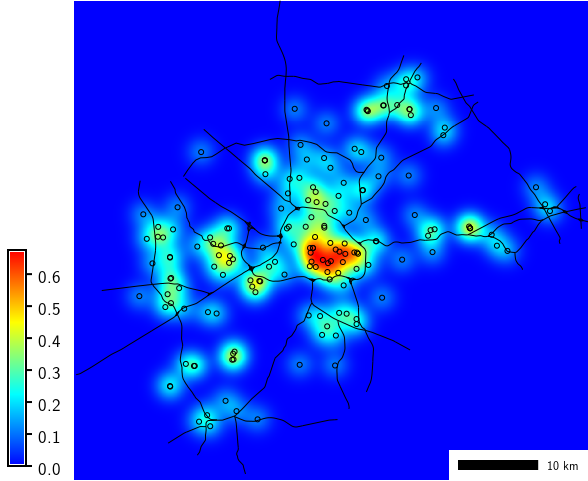## NAME

v.kernel - Generates a raster density map from vector points map.
Density is computed using a moving kernel. Optionally generates a vector density map on a vector network.

## KEYWORDS

vector, kernel density, point density, heatmap, hotspot

## SYNOPSIS

v.kernel
v.kernel --help
v.kernel [-oqnm] input=name [net=name] [output=name] [net_output=name] radius=float [dsize=float] [segmax=float] [distmax=float] [multiplier=float] [node=string] [kernel=string] [--overwrite] [--help] [--verbose] [--quiet] [--ui]

### Flags:

-o
Try to calculate an optimal radius with given 'radius' taken as maximum (experimental)
-q
Only calculate optimal radius and exit (no map is written)
-n
In network mode, normalize values by sum of density multiplied by length of each segment. Integral over the output map then gives 1.0 * multiplier
-m
In network mode, multiply the result by number of input points
--overwrite
Allow output files to overwrite existing files
--help
Print usage summary
--verbose
Verbose module output
--quiet
Quiet module output
--ui
Force launching GUI dialog

### Parameters:

input=name [required]
Name of input vector map with training points
net=name
Name of input network vector map
output=name
Name for output raster map
net_output=name
Name for output vector density map
Outputs vector map if network map is given
dsize=float
Discretization error in map units
Default: 0.
segmax=float
Maximum length of segment on network
Default: 100.
distmax=float
Maximum distance from point to network
Default: 100.
multiplier=float
Multiply the density result by this number
Default: 1.
node=string
Node method
Options: none, split
Default: none
none: No method applied at nodes with more than 2 arcs
split: Equal split (Okabe 2009) applied at nodes
kernel=string
Kernel function
Options: uniform, triangular, epanechnikov, quartic, triweight, gaussian, cosine
Default: gaussian

## DESCRIPTION

v.kernel generates a raster density map from vector points data using a moving kernel. Available kernel density functions are uniform, triangular, epanechnikov, quartic, triweight, gaussian, cosine, default is gaussian.

The module can also generate a vector density map on a vector network. Conventional kernel functions produce biased estimates by overestimating the densities around network nodes, whereas the equal split method of Okabe et al. (2009) produces unbiased density estimates. The equal split method uses the kernel function selected with the kernel option and can be enabled with node=split.

## NOTES

The multiplier option is needed to overcome the limitation that the resulting density in case of a vector map output is stored as category (integer). The density result stored as category may be multiplied by this number.

For the gaussian kernel, standard deviation for the gaussian function is set to 1/4 of the radius.

With the -o flag (experimental) the command tries to calculate an optimal radius. The value of radius is taken as maximum value. The radius is calculated based on the gaussian function, using ALL points, not just those in the current region.

## EXAMPLES

Compute density of points (using vector map of schools from North Carolina sample dataset):
```g.region region=wake_30m
r.colors map=schools_density color=bcyr
```School density

## KNOWN ISSUES

The module only considers the presence of points, but not (yet) any attribute values.

## REFERENCES

• Okabe, A., Satoh, T., Sugihara, K. (2009). A kernel density estimation method for networks, its computational method and a GIS-based tool. International Journal of Geographical Information Science, Vol 23(1), pp. 7-32.
DOI: 10.1080/13658810802475491

v.surf.rst

Overview: Interpolation and Resampling in GRASS GIS

## AUTHORS

Stefano Menegon, ITC-irst, Trento, Italy